# Isotoxal figure

In geometry, a polytope (for example, a polygon or a polyhedron), or a tiling, is isotoxal or edge-transitive if its symmetries act transitively on its edges. Informally, this means that there is only one type of edge to the object: given two edges, there is a translation, rotation and/or reflection that will move one edge to the other, while leaving the region occupied by the object unchanged.

The term isotoxal is derived from the Greek τοξον meaning arc.

## Isotoxal polygons

An isotoxal polygon is an equilateral polygon, but not all equilateral polygons are isotoxal. The duals of isotoxal polygons are isogonal polygons.

In general, an isotoxal 2n-gon will have Dn (*nn) dihedral symmetry. A rhombus is an isotoxal polygon with D2 (*22) symmetry.

All regular polygons (equilateral triangle, square, etc.) are isotoxal, having double the minimum symmetry order: a regular n-gon has Dn (*nn) dihedral symmetry. A regular 2n-gon is an isotoxal polygon and can be marked with alternately colored vertices, removing the line of reflection through the mid-edges.

Example isotoxal polygons
D2 (*22) D3 (*33) D4 (*44) D5 (*55)
Rhombus Equilateral triangle Concave hexagon Self-intersecting hexagon Convex octagon Regular pentagon Self-intersecting (regular) pentagram Self-intersecting decagram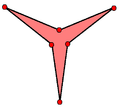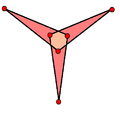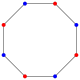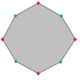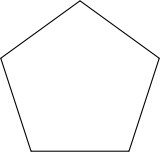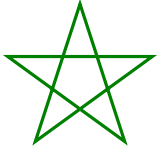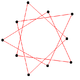## Isotoxal polyhedra and tilings

An isotoxal polyhedron or tiling must be either isogonal (vertex-transitive) or isohedral (face-transitive) or both.

Regular polyhedra are isohedral (face-transitive), isogonal (vertex-transitive) and isotoxal. Quasiregular polyhedra are isogonal and isotoxal, but not isohedral; their duals are isohedral and isotoxal, but not isogonal.

Examples
Quasiregular
polyhedron
Quasiregular dual
polyhedron
Quasiregular
star polyhedron
Quasiregular dual
star polyhedron
Quasiregular
tiling
Quasiregular dual
tiling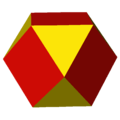A cuboctahedron is isogonal and isotoxal polyhedron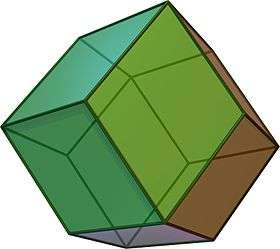A rhombic dodecahedron is an isohedral and isotoxal polyhedron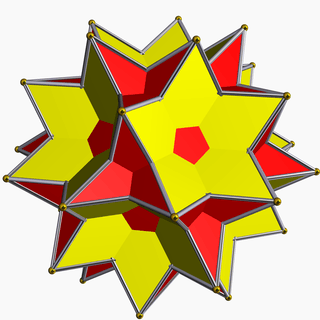A great icosidodecahedron is isogonal and isotoxal star polyhedron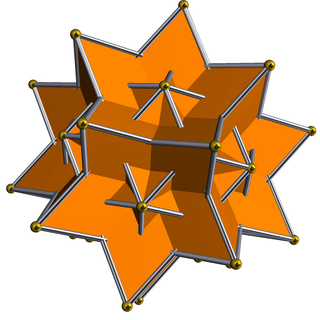A great rhombic triacontahedron is an isohedral and isotoxal star polyhedron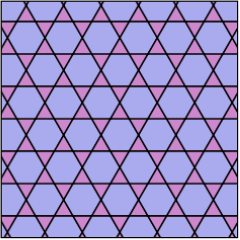The trihexagonal tiling is an isogonal and isotoxal tiling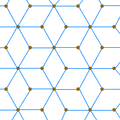The rhombille tiling is an isohedral and isotoxal tiling with p6m (*632) symmetry.

Not every polyhedron or 2-dimensional tessellation constructed from regular polygons is isotoxal. For instance, the truncated icosahedron (the familiar soccerball) has two types of edges: hexagon-hexagon and hexagon-pentagon, and it is not possible for a symmetry of the solid to move a hexagon-hexagon edge onto a hexagon-pentagon edge.

An isotoxal polyhedron has the same dihedral angle for all edges.

There are nine convex isotoxal polyhedra formed from the Platonic solids, 8 formed by the Kepler–Poinsot polyhedra, and six more as quasiregular (3 | p q) star polyhedra and their duals.

There are 5 polygonal tilings of the Euclidean plane that are isotoxal, and infinitely many isotoxal polygonal tilings of the hyperbolic plane, including the Wythoff constructions from the regular hyperbolic tilings {p,q}, and non-right (p q r) groups.